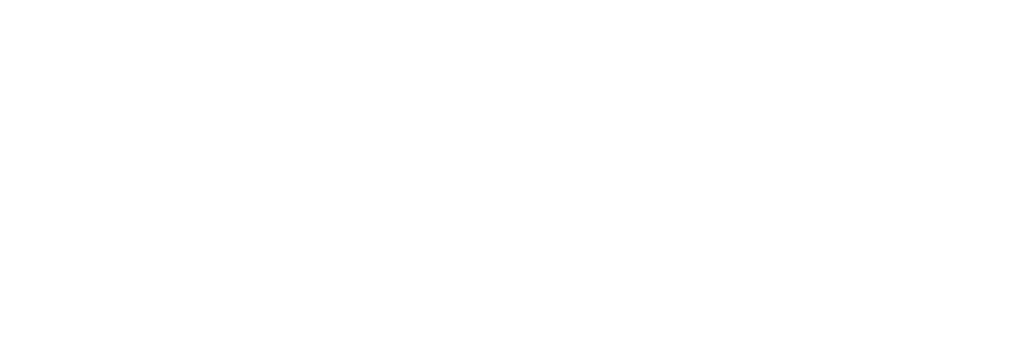# Whole Numbers Solution Chapter 2 Class 6 Maths

Whole Numbers Solution Chapter 2 Class 6 Maths Answer

1. Write the predecessor and successor of 19; 1997; 12000; 49; 100000.
2. Is there any natural number that has no predecessor?
3. Is there any natural number that has no successor? Is there a last natural number?

1.

19

predecessor =

successor =

• 1997

predecessor = 1997 – 1 = 1996

successor = 1997 +1 = 1998

• 12000

predecessor = 12000 – 1 =11999

successor = 12000 +1 = 12001

• 49

predecessor = 49 – 1 =48

successor = 49 + 1 = 50

• 100000

predecessor = 100000 – 1 = 99999

successor = 100000 + 1 = 100001

2. 1 (one): Predcessor of 1 is zero which is not a natural number  . So, 1 do not have a natural predecessor.

1. Are all natural numbers also whole numbers?
2. Are all whole numbers also natural numbers?
3. Which is the greatest whole number?

1. Yes. Zero & natural numbers = whole number

2. No. Zero is not a natural number.

3. Infinity